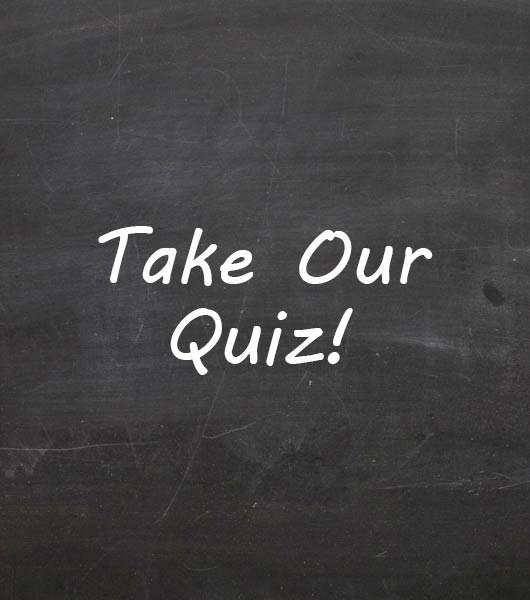Simple Machines Assessment Mcfadden Period 2

43 Questions | Total Attempts: 36Settings• 1.
A slanted surface used to raise an object is ______________
• A.

An inclined plane

• B.

A screw

• C.

An effort ramp

• D.

An efficiency board

• 2.
A device that does work with only one movement and changes the size and direction of a force is __________________
• A.

A compound machine

• B.

A simple machine

• C.

An effort machine

• D.

A wedge

• 3.
A bar that is free to pivot about a fixed point is a _____________
• A.

Lever

• B.

Wedge

• C.

Fulcrum

• D.

Ramp

• 4.
The work output of a machine compared to the work input is the _________ of the machine.
• A.

Efficiency

• B.

Power

• C.

Effort

• D.

Resistance

• 5.
The amount by which a machine multiplies an effort force is called the _________
• A.

Resistance force

• B.

Efficiency

• C.

Fulcrum

• D.

• 6.
Two inclined planes put together form a simple machine called  _________________
• A.

A screw

• B.

A wedge

• C.

A lever

• D.

An incline plane

• 7.
An inclined plane wrapped around a cylindrical post is a
• A.

Screw

• B.

Wedge

• C.

Lever

• D.

Block and tackle

• 8.
A winding mountain road is an example of what kind of simple machine?
• A.

A lever

• B.

An inclined plane

• C.

A wheel and axle

• D.

A pulley

• 9.
Two or more simple machines working together are called ________________
• A.

A pulley

• B.

A compound machine

• C.

A wheel and axle

• D.

A lever

• 10.
The unit for power is the ___________
• A.

Joule

• B.

MA

• C.

Watt

• D.

Second

• 11.
A lever with a mechanical advantage greater than 1 is used to _____________
• A.

Increase distance

• B.

Change direction

• C.

Decrease effort force

• D.

Increase speed

• 12.
Three of the listed simple machines are basically the same.  Choose the one that DOES NOT belong with the group.
• A.

Wedge

• B.

Lever

• C.

Pulley

• D.

Wheel and axle

• 13.
A system of pulleys designed to reduce the effort force is called _________________
• A.

A simple pulley

• B.

A fixed pulley

• C.

A movable pulley

• D.

A block and tackle

• 14.
Two simple machines that are part of a bicycle are _____________
• A.

An inclined plane and a wedge

• B.

An inclined plane and a lever

• C.

A gear and a wheel and axle

• D.

A wheel and axle and an inclined plane

• 15.
Which of the following groups of simple machines represent variations of the lever?
• A.

Pulley, inclined plane, and wedge

• B.

Pulley, wheel and axle

• C.

Pulley, wedge, and screw

• D.

Wedge, screw, and wheel and axle

• 16.
The MA of a single fixed pulley is always one because
• A.

The pulley changes only the direction of the effort force

• B.

The pulley changes only the size of the effort force

• C.

The pulley changes the size and direction of the effort force

• D.

The pulley changes only the speed at which the effort force is applied

• 17.
Which of the following CANNOT be done by a simple machine?
• A.

Change the direction of a force

• B.

Increase the amount of work being done

• C.

Decrease the time it takes to do the work

• D.

Transfer energy from one location to another

• 18.
A machine that changes only the direction of a force has an MA of ______________
• A.

10

• B.

5

• C.

3

• D.

1

• 19.
What is the IMA of the inclined plane below?IMA = Effort Distance/Resistance DistanceActual MA = Resistance Force/ Effort ForceW = FxDWin = Fe x DeWout = Fr x DrWin = WoutEfficiency = Wout/Win x 100
• A.

1

• B.

3

• C.

.33

• D.

5

• 20.
What is the IMA of the pulley system below?
• A.

1

• B.

3

• C.

6

• D.

12

• 21.
What is the IMA for this pulley system?
• A.

0

• B.

2

• C.

4

• D.

6

• 22.
Which pulley system will require the greatest effort distance to raise the resistance force 1 meter?
• A.

System A

• B.

System B

• C.

The effort distance will be the same for both A and B

• D.

• 23.
Which lever below is a first class lever?
• A.

Lever A

• B.

Lever B

• C.

Lever C

• 24.
Which lever below is a second class lever?
• A.

Lever A

• B.

Lever B

• C.

Lever C

• 25.
Which lever below is a third class lever?
• A.

Lever A

• B.

Lever B

• C.

Lever CBack to top